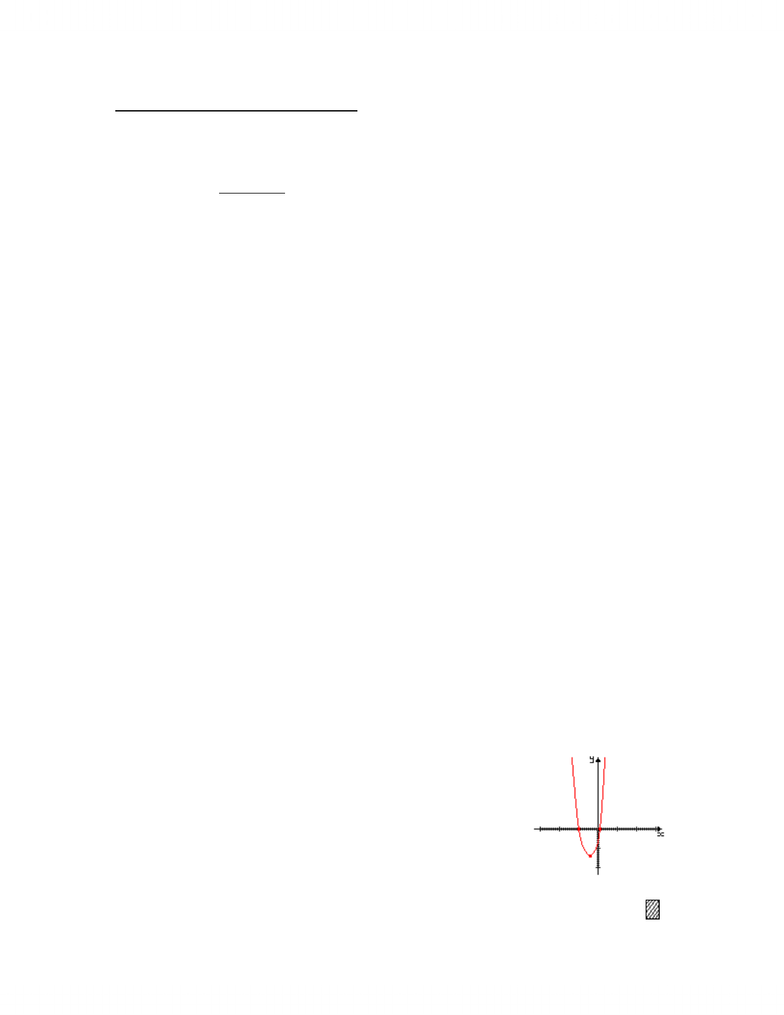# Just about everything Prof Grinnell wrote on the board.

24 views3 pages
School
Department
Course09/17/10
Review of Functions + Other Basics
(§ 2.1-2.5, 4.1-4.4)
Ex 1 Let p(x) = 4x2 + 3x - 1
Evaluations: p(0) = -1
p(3) = 36 + 9 - 1 = 44
p(x + 2) vs. p(x) + 2
= 4(x + 2)2 + 3(x + 2) ² 1 = 4x2+3x+1
= 4(x2 + 4x + 4) + 3x + 6 - 1
= 4x2 + 16x + 16 + 3x + 6 - 1
= 4x2 + 19x + 21 * in descending powers
Find a function g such that g(x + 2) = p(x) = 4x2 + 3x ² 1
/HVWU\JRIWKHIRUPJ[ D[2 + bx + c
We solve for a, b, c
a(x + 2)2 + b(x + 2) + c = 4x2 + 3x - 1
ax2 + 4ax + 4a + bx + 2b + c = 4x2 + 3x ² 1
, · , a = 4
16 + b = 3
b = -13
You check that c=9.
E´&RPSOHWHWKHVTXDUHµLVDVWRZULWHS[LQWKHIRUP
y = p(x) = a(x + h)2 + k where a, h, k are constants.
p(x) = 4x2 + 3x ² 1
= 4(x2 + 3/4 x) ² 1
= 4(x2 + 3/4 x + 9/64 - 9/64) ² 1
= 4(x2 + 3/8 x)2 ² 19/16 ² 16/16
= 4(x2 + 3/8 x)2 ² 25/16
¶D K3/8, k = -25/16
Domain of p is all real numbers (i.e., R or (-
Vertex is the point (-3/8 , -25/16 ).
Parabola opens up.
Quadratic Formula givers p(x) = 0 when x = -1, 1/9.
www.notesolution.com
Unlock document

This preview shows page 1 of the document.
Unlock all 3 pages and 3 million more documents.

## Get access

\$10 USD/m
Billed \$120 USD annuallyHomework Help
Study Guides
Textbook Solutions
Class Notes
Textbook Notes
Booster Class